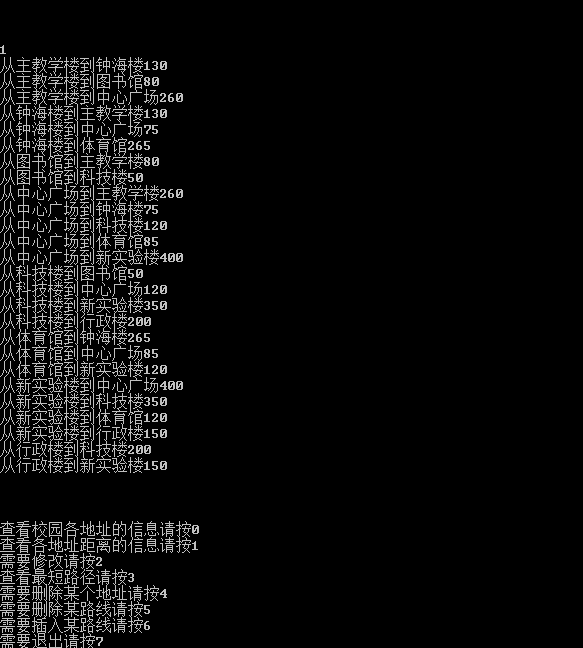# 课程设计

《数据结构课程设计》

 课程题目 校园地图 课程编号 学生姓名 姚文华 所在专业 物联网工程 所在班级 物联1131 任课老师 易学明 实习时间 设计成绩 老师评语

GDOU是真是一个好地方，校园如一座大花园，美丽而宽广。校园有许多建筑如教学楼、饭堂、宿舍楼、图书馆、体育馆、运动场、商业街、医院等，还有一些著名的风景点。现请根据学校的平面图，找出一些重要的场所，画出学校的平面图（场所可以根据其重要性适当减少），根据实际画出不同点间的路径，并估算每两个场所间的路径长。请设计数据结构并编程，当给出一个出发点和要到达另外一个场所的信息时，请给出最佳路径，并输出路径相关信息。

1、独立完成，设计算法并编写代码，调试通过。

2、写设计说明书。

3、以个人独立完成。每一个选择一个题目。选题方式是：自己学号整除5所得的余数是几就做几号题。如学号为12做2号题，学号为5的做0号题。

4、时间：从第13周开始收集资料，进行准备。具体设计时间在16-17周（等实验室安排）。在设计周周五检查（在机房子单独接受老师检查并提问），次周周五前提交设计说明书（实习报告）。

5、实习单独计算成绩，学分1分，成绩好坏和考试没关系。

1、问题描述

a)     顶点数

b)     边数

c)     边的长度

2、功能需求

要求完成以下功能：

a)       输出顶点信息：将校园内各位置输出。

b)       输出边的信息：将校园内每两个位置（若两个位置之间有直接路径）的距离输出。

c)       修改：修改两个位置（若两个位置之间有直接路径）的距离，并重新输出每两个位置（若两个位置之间有直接路径）的距离。

d)       求最短路径：输出给定两点之间的最短路径的长度及途径的地点或输出任意一点与其它各点的最短路径。

e)       删除：删除任意一条边。

f)        插入：插入任意一条边。

3、实现要点

a)  对图的创建采用邻接矩阵的存储结构，而且对图的操作设计成了模板类。为了便于处理，对于图中的每一个顶点和每一条边都设置了初值。

b)  为了便于访问，用户可以先输出所有的地点和距离。

c)  用户可以随意修改两点之间好的距离。

d)  用户可以增加及删除边。

e)  当用户操作错误时，系统会出现出错提示。

1.       抽象数据类型图的定义如下：

R={VR}

VR={（v，w）| v , w∈V, (v , w)表示v和w之间存在路径}

CreatGraph(&G, V, VR)

DestroyGraph(&G)

LocateVex(G, u)

GetVex(G, v)

InsertVex(&G, v)

DeleteVex(&G, v)

InsertArc(&G, v, w)

DeleteArc(&G, v, w)

2.       主程序

void main()

{

初始化；

while(“命令”!=“退出”)

{

Switch语句

接受命令（输入选择项序号）；

处理命令；

}

}

3.       本程序运用函数的调用，只有两个模块，它们的调用关系为：

#include <stdio.h>

#include <iostream.h>

#include<stdlib.h>

#include<conio.h>

#include <malloc.h>

#include<string.h>

#define MAX 10000

#define MAXLEN 8

typedef struct                            //图中顶点表示点，存放点名称

{

char name;

int num;

}VEXTYPE;

typedef struct

{

VEXTYPE vexs[MAXLEN];               //顶点的信息

int vexnum,arcnum ;                      //顶点数和边数

}MGraph;

MGraph b;

MGraph InitGraph()

{                                      /*建立无向网的邻接矩阵结构*/

int i, j;

MGraph G;

G.vexnum =8;                            //存放顶点数

G.arcnum =13;                           //存放边点数

for(i=0;i<G.vexnum;i++)

G.vexs[i].num=i;

strcpy(G.vexs.name,"主教学楼");

strcpy(G.vexs.name,"钟海楼");

strcpy(G.vexs.name,"图书馆");

strcpy(G.vexs.name,"中心广场");

strcpy(G.vexs.name,"科技楼");

strcpy(G.vexs.name,"体育馆");

strcpy(G.vexs.name,"新实验楼");

strcpy(G.vexs.name,"行政楼");

for(i=0;i<G.vexnum;i++)

for(j=0;j<G.vexnum;j++)

G.arcs[i][j]=MAX;

G.arcs=130;

G.arcs=80;

G.arcs=260;

G.arcs=75;

G.arcs=50;

G.arcs=120;

G.arcs=265;

G.arcs=85;

G.arcs=400;

G.arcs=350;

G.arcs=120;

G.arcs=200;

G.arcs=150;

for(i=0;i<G.vexnum;i++)

for(j=0;j<G.vexnum;j++)

G.arcs[j][i]=G.arcs[i][j];

return G;

}

{

cout<<endl;

cout<<endl;

cout<<endl;

cout<<"查看校园各地址的信息请按0\n";

cout<<"查看各地址距离的信息请按1\n";

cout<<"需要修改请按2\n";

cout<<"查看最短路径请按3\n";

cout<<"需要删除某个地址请按4\n";

cout<<"需要删除某路线请按5\n";

cout<<"需要插入某路线请按6\n";

cout<<"需要退出请按7\n";

cout<<endl;

cout<<endl;

cout<<endl;

}

void PutOutVex(MGraph *G)                 //输出每个顶点的信息

{

int v;

for(v=0;v<G->vexnum;v++)

cout<<G->vexs[v].num<<G->vexs[v].name<<endl;

}

void PutOutArc(MGraph *G)                 //输出每条边的信息

{

for(int i=0;i<G->vexnum;i++)

for(int j=0;j<G->vexnum;j++)

if(G->arcs[i][j]<MAX)

{cout<<"从"<<G->vexs[i].name<<"到"<<G->vexs[j].name<<G->arcs[i][j]<<endl;

}

}

void Change(MGraph *G)                    //修改

{ int v0,v1,length;

cout<<"change\n";

cin>>v0;

cin>>v1;

cout<<"length:";

cin>>length;

G->arcs[v0][v1]=G->arcs[v1][v0]=length;

}

void Dijkstra(MGraph * G)                  //迪杰斯特拉算法求最短路径

{

intv,w,i,min,t=0,x,v0,v1;

int final,D, p;

cout<<"请输入起点位置的编号:\n";

cin>>v0;

if(v0<0||v0>G->vexnum)

{

cout<<"此点编号不存在!请重新输入地址编号:";

cin>>v0;

}

cout<<"请输入终点位置编号:\n";

cin>>v1;

if(v1<0||v1>G->vexnum)

{

cout<<"此点编号不存在!请重新输入顶点编号:";

cin>>v1;

}

for(v=0;v<G->vexnum;v++)

{// 初始化final,p,final[v]=1即已经求得v0到v的最短路径，

//p[v][w]=1则是w从v0到v当前求得最短路径上的顶点，D[v]带权长度

final[v]=0;

D[v]=G->arcs[v0][v];

for(w=0;w<G->vexnum;w++)

p[v][w]=0;

if(D[v]<MAX)

{

p[v][v0]=1;p[v][v]=1;

}

}

D[v0]=0;final[v0]=1;

for(i=1;i<G->vexnum;i++)

{

min=MAX;

for(w=0;w<G->vexnum;w++)

if(!final[w])

if(D[w]<min){v=w;min=D[w];}

final[v]=1;

for(w=0;w<G->vexnum;w++)

if(!final[w]&&(min+G->arcs[v][w]<D[w]))

{

D[w]=min+G->arcs[v][w];

for(x=0;x<G->vexnum;x++)

p[w][x]=p[v][x];

p[w][w]=1;

}

}

cout<<"从"<<G->vexs[v0].name<<"到"<<G->vexs[v1].name<<"的最短路径长度为："<<D[v1]<<endl;

cout<<"路径为：";

for(intj=0;j<G->vexnum;j++)

{

if(p[v1][j]==1)

cout<<G->vexs[j].name<<endl;

}

}

void DeleteVex(MGraph *G)                  //删除某个顶点

{

int row,col;

int v0;

cout<<"请输入要删除的顶点";

cin>>v0;

for(inti=v0;i<G->vexnum;i++)

G->vexs[i]=G->vexs[i+1];

G->vexnum--;

for(row=0;row<G->vexnum;row++)

{

for(col=v0;col<G->vexnum;col++)

G->arcs[row][col]=G->arcs[row][col+1];

}

for(col=0;col<G->vexnum;col++)

{

for(row=v0;row<G->vexnum;row++)

G->arcs[col][row]=G->arcs[col][row+1];

}

}

void DeleteArc(MGraph *G)                  //删除某条边

{

int v0,v1;

cout<<"请输入两顶点:\n";

cin>>v0>>v1;

G->arcs[v0][v1]=MAX;

G->arcs[v1][v0]=MAX;

}

void InsertArc(MGraph *G)                  //插入某条边

{

int v0,v1,l=0;

cout<<"请输入两地址编号:\n";

cin>>v0>>v1;

cout<<"请输入路径长度:\n";

cin>>l;

G->arcs[v0][v1]=l;

G->arcs[v1][v0]=l;

}

void main()                                //主函数

{ int a;

b=InitGraph();

cin>>a;

while(a!=7)

{

switch(a)

{

case7:exit(1);break;

default:break;

}

cin>>a;

}

}一直以来这方面就不是我的强项，所以借这次机会我又好好的学习了一次，发现了自己的不足之处，以前基本上都是照着书上的打代码，现在要自己设计还是有很大的困难，但是这次通过自己的学习和请教同学也学到了很多，希望以后还会有这样的机会，以后也相信自己会学的更加的好！

©️2019 CSDN 皮肤主题: 大白 设计师: CSDN官方博客